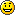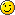# 提高Monte Carlo模拟定积分计算的精度

• 平均值方法是根据弱大数定律证明得来的。构造如此

均方误差

而由$Schwartz$不等式知

,从中便可知 $MC$ 法具有半阶收敛性，且收敛阶不依赖问题的维数，这样在计算高维积分的时候，比较优越。但是收敛速度还是很慢的，是一个比较坏的收敛速度。因此在有其他高效率、高精度的确定型算法时，就不要采用$MC$法，那是在没有更好的算法时更好的抉择，比如超高维积分。
• 由均方误差可知，要提高 $MC$ 法的精确度，那么久必须得增大$N$，减小$VAR(f)$，也就是说，要增加样本量，同时改善抽样方法的选择，这时便有很多方法选择，书中提到了四种方法，我就谈谈我的理解和试验。
1. 分层抽样。核心就是将原来的 $[0,1]$ 区间 $M$ 等分后在各个小区间上分别再以均匀分布抽样 $N/M$ 个点，然后以

理论证明知这样方差减少，具体证明式子较多就不再写，否则就是一本教材的翻版了。
模拟$\int_{0}^{1}exp(-x^2/2)/\sqrt{2pi}dx$,具体实验结果如下

 1 2 3 4 5 6 7  M<-100 N<-1000 A=matrix(as.numeric(gl(M,N/M))-1,ncol=N/M,byrow=T) B=replicate(M,runif(N/M)) x=1/M*t(B)+A/M y=exp(-x^2/2)/sqrt(2*pi) I=mean(y)

结果为0.3413287，可知只取了1000个样本点的情况下，精确度已经十分好了，而一般的均值模拟
达到此精确度则需要$10^7$个点。

2. 对偶变量法。
这是一种特殊的针对于定义域具有一定对称性的函数性质的技巧。基于这样的命题:如果$f(x)$是单调的，则$Cov(f(X),f(1-X))\leq0$,这里$X\sim U[0,1]$。则有

,
$EI_N=I(f)$,
经过证明知 $Var(I_N)\leq \frac{1}{2N}Var(f)$，方差显然减少。

但是这种方法只是对于定义域有一定对称性的函数，如果对一种函数先验了解不多，而且对称性不知，则运用会出现失误，所以具有一定局限性。
模拟$\int_{0}^{1}exp(-x^2/2)/\sqrt{2pi}dx$的代码如下：

 1 2 3  a=runif(10^6) y=exp(-a^2/2)/sqrt(2*pi)+exp(-(1-a)^2/2)/sqrt(2*pi) b=sum(y)/(2*10^6)

结果为 0.3413487，精确度也得到了提高。

3. 重要性抽样：核心就是构造一个新的积分

其中$p(x)$$[0,1]$的概率密度，$\int_{0}^{1}p(x)dx=1$,$p(x)\geq 0$。这样得到类似于最初最简单的那种均值法的新的形式积分近似$I(f)\approx 1/N\sum_{i=1}^{1}f(Y_i)/p(Y_i)$$Y_i$为遵循p(y)的独立同分布随机变量(i.i.d),而之所以这样构造，可以根据这幅图来理解，$\int_{0}^{a}+\int_{b}^{1}f(x)dx$$I(f)$ 很少比例(即图像的边缘部分)，尽可能的不让随机点去计算这些值，这样的“拟合”积分较好（面积）,否则很多的随机点在 $[a,b]$ 外，为计算小比例的东西做贡献，这样必定精度较差，所以我们要期望尽可能的值出现在峰值处。我们称其为“重要性”抽样。理论分析，

,

.
只要选取适当的 $p(y)$ 就可以使方差减少。
当然不可能是$Var(f/p)=0$，这在图的模拟便可知，$p(y)$不可能与$f(x)$相合的同时，还有$\int_{0}^{1}p(x)dx=1$。但是$p$难以选择，需要对问题先验有了一定的了解程度才能适当选取。比如对于正态分布求$\int_{0}^{1}exp(-x^2/2)/\sqrt(2pi)dx$，我不太好选$p$，就没有做相关的模拟了。
4. 控制变量法。
核心也是构造，数学式子$I_N(f)=i/N\sum_{i=1}^{N}(f-g)(X_i)+I(g)$.
需要$I(g)$已知，但是这是同重要性抽样一样，都不知道，也需要对问题先验有了一定的了解程度，才能好的选择，使$I(g)$已知,并且有$Var(f-g)\leq Var(f)$。相关模拟我也没有再做

1. 虽说 I can't get all of it, 大概能理解个六七分吧 有为青年啊 .. 好好修改充实一下投稿到谢老大的地盘吧? 有些地方写得有点过于简略了 我这种学术功底浅浅的人读起来困难啊部分LaTeX公式有点瑕疵 比如少一半括号之类的.

WP CodeBox 没给你装? 没有的话搜一个装上.
用法:

代码

lang="LANGUAGE"：代码的语言 参照太云的用法 用Ruby的高亮方案就好
line="N"：开始行数
colla="+/-"： ”+“表示展开，”-“表示收缩

还有 我极度推荐扫一眼王垠的文章:
http://docs.huihoo.com/homepage/shredderyin/tex/tex_formula.html
《怎样输入完美的TeX公式》
公式还有改进的空间.
虽然我总是输入不完美的• 再次修改了一下，你可以再看下，应该不太和谐的地方少了不少吧...TeX公式那个很受用，不错不错

至于里面的内容，理论证明我是尽量简化了，不然会让文章很长，和本教材没有太大的区别。如果你想研究，可以借书给你看。

谢老大那里呀，水平不够还是不敢贸然前往，毕竟众人围观下，四目相望，惧之也...

• 我已经忘记是哪本书了。。三年多了...依稀记得当年看的貌似是这本书http://book.douban.com/subject/1811284/